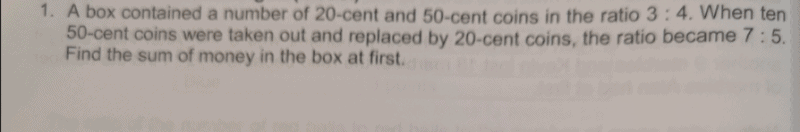# QuestionI took away 10 50-cent coins and replaced with 10 20-cent coins, and just couldn’t get the answer, until…

I took away 10 50-cent coins of value \$5, and replaced with equal value of 20-cent coins, (\$5 ÷ \$0.20) = 25 coins

 20-cent 50-cent Quantity at first 3u 4u Quantity change +25 -10 Quantity in the end 7p = 3u + 25 5p = 4u – 10 Multiply by 5 Multiply by 7 35p = 15u + 125 35p = 28u – 70

28u – 70 = 15u + 125

13u = 195

1u = 15

Value of 20-cent coins at first -> 3u × \$0.20 = \$9

Value of 50-cent coins at first -> 4u × \$0.50 = \$30

Sum of money in the box -> \$9 + \$30 = \$39